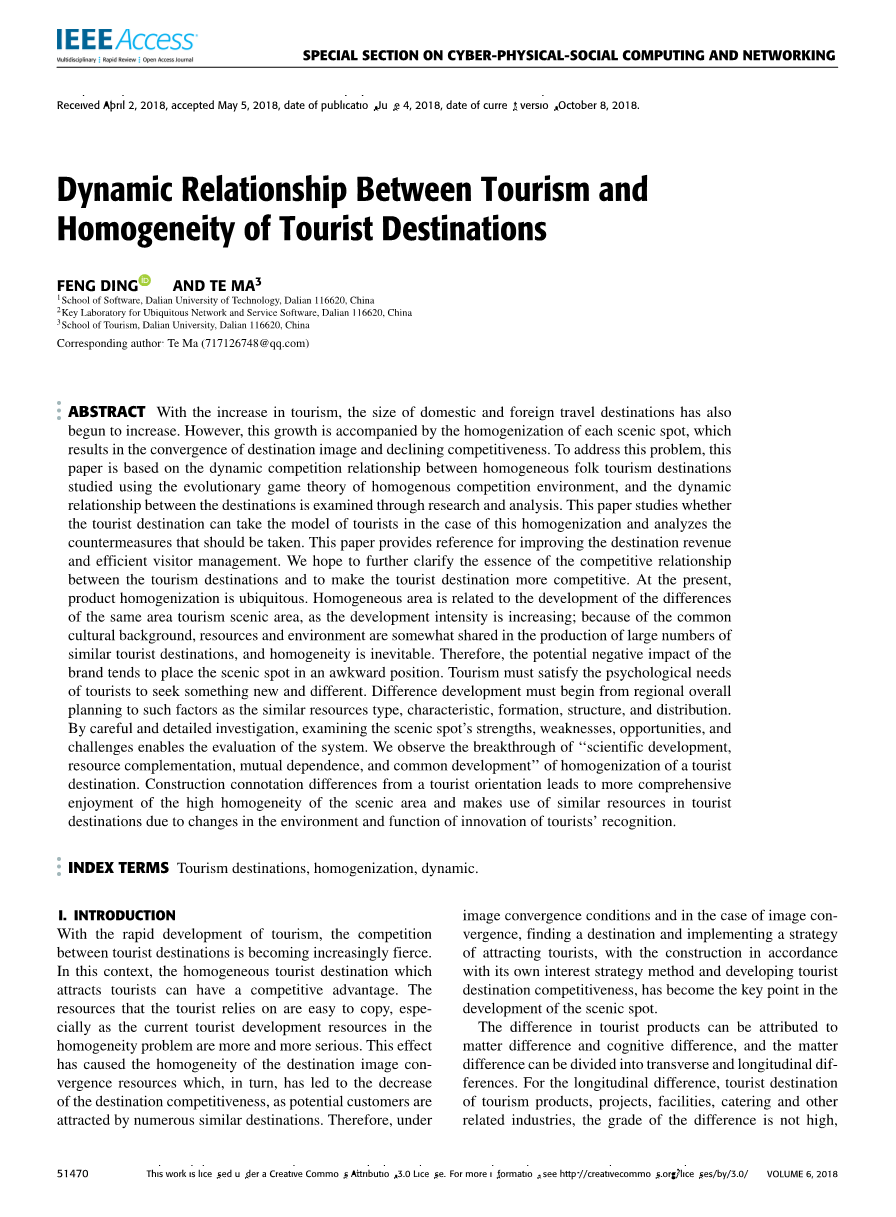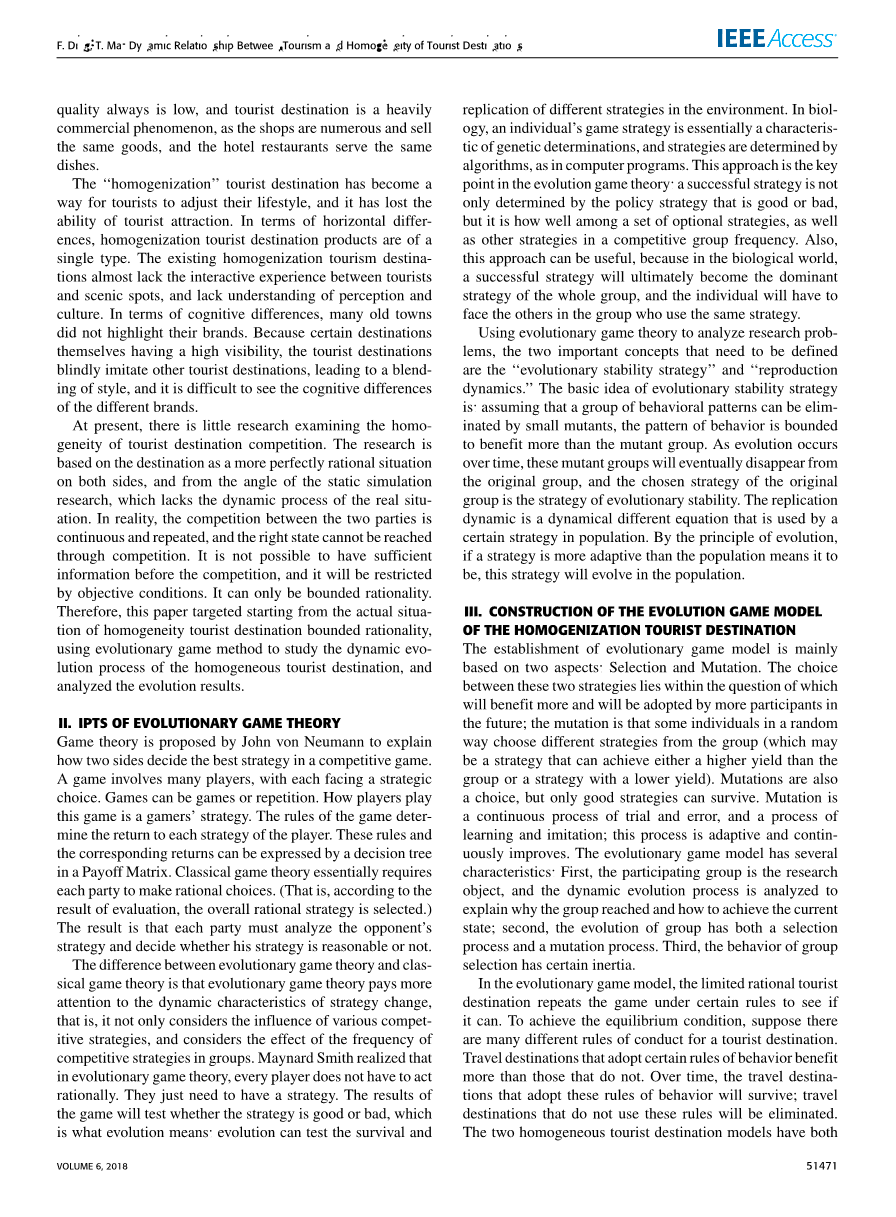# 旅游业与旅游业的动态关系旅游目的地同质化外文翻译资料

2021-11-26 10:11“同质化”旅游目的地已成为旅游者调整生活方式的一种方式，丧失了旅游吸引力。从横向差异来看，同质化旅游目的地产品属于单一类型。现有的同质化旅游目的地几乎缺乏旅游者与景区之间的互动体验，缺乏对感知和文化的理解。在认知差异方面，许多老城区并没有突出他们的品牌。由于某些旅游目的地本身具有较高的知名度，旅游目的地盲目模仿其他旅游目的地，导致风格的融合，很难看到不同品牌的认知差异。

（1）游戏只有两个方面：旅游目的地A和B，两者都有合理的界限。

（2）行为策略：A、B两个旅游目的地将根据各自的收益采用不同的策略。研究模拟了游客的旅游目的地。

（3）1minus;行为策略采用概率：在旅游目的地A和B的竞争中，假设A获得游客的概率为：X，未获得游客的概率为：B获得游客的概率为Y，未获得游客的概率为1minus;XY

（4）参数假设及基本解释：M1：旅游目的地不捕捉游客的利益；M2、M3：一个目的地一个得到，一个不得到。m2为游客后目的地的收益；m3为目的地的收益；m4。旅游目的地得到旅游者的利益；c：旅游者潜在风险的损失。因此，有m2lt; M4 lt; M1 lt; M3

（5）返回矩阵：根据上述假设，得到目的地A、B动态博弈的收益矩阵（如表1所示）。

 竞争的两方 游客B目的地 可获得 不可获得 游客A目的地 可获得 不可获得

EAY = Ym4 (1 minus; Y)m2 (1)

EAN = Y(m3 minus; c) (1 minus; Y)(m1 minus; c) (2)

EA = XEAY (1 minus; X)EAN = XYm4 X(1 minus; Y)m2

Y(1 minus; X)(m3 minus; c) (1 minus; Y)(1 minus; X)(m1 minus; c) (3)

dX/dt = X(EAY minus; EA) = X(1 minus; X)[m2 c – m1]

Y (m4 m1 minus; m3 minus; m2)] (4)

dY/dt = Y (1 minus; Y )[m2 c minus; m1 X(m4 m1 minus; m3 minus; m2)] (5)

dX/dt = X(1 minus; X)[m2 c minus; m1 Y (m4 m1 minus; m3 minus; m2)]

dY/dt = Y (1 minus; Y )[m2 c minus; m1 X(m4 m1 minus; m3 minus; m2)]

A.旅游目的地分析

The F(X) = dX/dt , derivative F(X) of X: dF(X)/dX = (1minus;2X)[m2 c minus; m1 Y (m4 m1 minus; m3 minus; m2)]

1. 当 0 lt; （m1minus;m2minus;c） /（m1minus;m2 m4minus;m3） le; 1

（1）当Y =（m1minus;m2minus;c）/（m1minus;m2 m4minus;m3） ,对于所有X点都是稳定点，旅游目的地以不变的策略选择获得游客，并具有一个常数

（2）当Yne;（m1minus;m2minus;c）/（m1minus;m2 m4minus;m3），F(X)=0,我们得到X=0,X=1。当n Y gt; （m1minus;m2minus;c）/（m1minus;m2 m4minus;m3），那么 dF(X)/dX |X=0 gt; 0，dF(X)/dX |X=1 lt; 0，因为稳定点是X=1，因为稳定点是x=1、旅游目的地B的概率适应游客大于一定的价值，理性的A会选择一个旅游者的策略。当Y＜（m1minus;m2minus;c）/（m1minus;m2 m4minus;m3），那么dF(X)/dX |X=0lt; 0，dF(X)/dX |X=1 ＞ 0，所以x=0是稳定点。旅游目的地B适应游客的概率大于一定值；A可以选择不接受游客的策略。

2. 当 0 lt; （m1minus;m2minus;c） /（m1minus;m2 m4minus;m3） ＞ 1

F(X)=0, 让 X = 0, X = 1,但dF(X) /dX |X=0 lt; 0，dF(X)/dX |X=1 gt; 0, X=0是稳定点。A和B都选择了旅游者的策略，双方的收入都大于选择旅游者策略的收入。当双方都不为游客服务时，一方的利润大于双方同时获得的用户利益。此时，旅游目的地策略选择不吸引其他游客。

3. 当 0 lt; （m1minus;m2minus;c） /（m1minus;m2 m4minus;m3） ＜ 0

(1) 当 m1 minus; m2 minus; c gt; 0, dF(X)/dX |